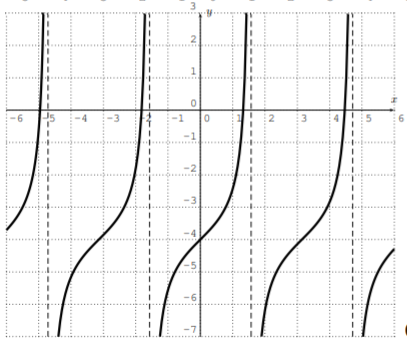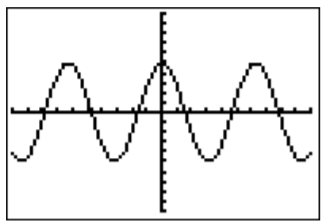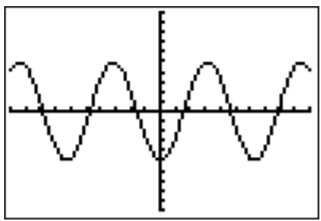# 17.3: Exercises

$$\newcommand{\vecs}{\overset { \rightharpoonup} {\mathbf{#1}} }$$ $$\newcommand{\vecd}{\overset{-\!-\!\rightharpoonup}{\vphantom{a}\smash {#1}}}$$$$\newcommand{\id}{\mathrm{id}}$$ $$\newcommand{\Span}{\mathrm{span}}$$ $$\newcommand{\kernel}{\mathrm{null}\,}$$ $$\newcommand{\range}{\mathrm{range}\,}$$ $$\newcommand{\RealPart}{\mathrm{Re}}$$ $$\newcommand{\ImaginaryPart}{\mathrm{Im}}$$ $$\newcommand{\Argument}{\mathrm{Arg}}$$ $$\newcommand{\norm}{\| #1 \|}$$ $$\newcommand{\inner}{\langle #1, #2 \rangle}$$ $$\newcommand{\Span}{\mathrm{span}}$$ $$\newcommand{\id}{\mathrm{id}}$$ $$\newcommand{\Span}{\mathrm{span}}$$ $$\newcommand{\kernel}{\mathrm{null}\,}$$ $$\newcommand{\range}{\mathrm{range}\,}$$ $$\newcommand{\RealPart}{\mathrm{Re}}$$ $$\newcommand{\ImaginaryPart}{\mathrm{Im}}$$ $$\newcommand{\Argument}{\mathrm{Arg}}$$ $$\newcommand{\norm}{\| #1 \|}$$ $$\newcommand{\inner}{\langle #1, #2 \rangle}$$ $$\newcommand{\Span}{\mathrm{span}}$$$$\newcommand{\AA}{\unicode[.8,0]{x212B}}$$

## Exercise $$\PageIndex{1}$$

Find $$\sin(x)$$, $$\cos(x)$$, and $$\tan(x)$$ for the following angles.

1. $$x=120^\circ$$
2. $$x=390^\circ$$
3. $$x=-150^\circ$$
4. $$x=-45^\circ$$
5. $$x=1050^\circ$$
6. $$x=-810^\circ$$
7. $$x=\dfrac{5\pi}{4}$$
8. $$x=\dfrac{5\pi}{6}$$
9. $$x=\dfrac{10\pi}{3}$$
10. $$x=\dfrac{15\pi}{2}$$
11. $$x=\dfrac{-\pi}{6}$$
12. $$x=\dfrac{-54\pi}{8}$$
1. $$\sin \left(120^{\circ}\right)=\dfrac{\sqrt{3}}{2}, \cos \left(120^{\circ}\right)=-\dfrac{1}{2}, \tan \left(120^{\circ}\right)=-\sqrt{3}$$
2. $$\sin \left(390^{\circ}\right)=\dfrac{1}{2}, \cos \left(390^{\circ}\right)=\dfrac{\sqrt{3}}{2}, \tan \left(390^{\circ}\right)=\dfrac{\sqrt{3}}{3}$$
3. $$\sin \left(-150^{\circ}\right)=-\dfrac{1}{2}, \cos \left(-150^{\circ}\right)=-\dfrac{\sqrt{3}}{2}, \tan \left(-150^{\circ}\right)=\dfrac{\sqrt{3}}{3}$$
4. $$\sin \left(-45^{\circ}\right)=-\dfrac{\sqrt{2}}{2}, \cos \left(-45^{\circ}\right)=\dfrac{\sqrt{2}}{2}, \tan \left(-45^{\circ}\right)=-1$$
5. $$\sin \left(1050^{\circ}\right)=-\dfrac{1}{2}, \cos \left(1050^{\circ}\right)=\dfrac{\sqrt{3}}{2}, \tan \left(1050^{\circ}\right)=-\dfrac{\sqrt{3}}{3}$$
6. $$\sin \left(-810^{\circ}\right)=-1, \cos \left(-810^{\circ}\right)=0, \tan \left(-810^{\circ}\right)$$ is undefined
7. $$\sin \left(\dfrac{5 \pi}{4}\right)=-\dfrac{\sqrt{2}}{2}, \cos \left(\dfrac{5 \pi}{4}\right)=-\dfrac{\sqrt{2}}{2}, \tan \left(\dfrac{5 \pi}{4}\right)=1$$
8. $$\sin \left(\dfrac{5 \pi}{6}\right)=\dfrac{1}{2}, \cos \left(\dfrac{5 \pi}{6}\right)=-\dfrac{\sqrt{3}}{2}, \tan \left(\dfrac{5 \pi}{6}\right)=-\dfrac{\sqrt{3}}{3}$$
9. $$\sin \left(\dfrac{10 \pi}{3}\right)=-\dfrac{\sqrt{3}}{2}, \cos \left(\dfrac{10 \pi}{3}\right)=-\dfrac{1}{2}, \tan \left(\dfrac{10 \pi}{3}\right)=\sqrt{3}$$
10. $$\sin \left(\dfrac{15 \pi}{2}\right)=-1, \cos \left(\dfrac{15 \pi}{2}\right)=0, \tan \left(\dfrac{15 \pi}{2}\right)$$ is undefined
11. $$\sin \left(\dfrac{-\pi}{6}\right)=-\dfrac{1}{2}, \cos \left(\dfrac{-\pi}{6}\right)=\dfrac{\sqrt{3}}{2}, \tan \left(\dfrac{-\pi}{6}\right)=-\dfrac{\sqrt{3}}{3}$$
12. $$\sin \left(\dfrac{-54 \pi}{8}\right)=-\dfrac{\sqrt{2}}{2}, \cos \left(\dfrac{-54 \pi}{8}\right)=-\dfrac{\sqrt{2}}{2}, \tan \left(\dfrac{-54 \pi}{8}\right)=1$$

## Exercise $$\PageIndex{2}$$

Graph the function, and describe how the graph can be obtained from one of the basic graphs $$y=\sin(x)$$, $$y=\cos(x)$$, or $$y=\tan(x)$$.

1. $$f(x)=\sin(x)+2$$
2. $$f(x)=\cos(x-\pi)$$
3. $$f(x)=\tan(x)-4$$
4. $$f(x)=5\cdot \sin(x)$$
5. $$f(x)=\cos(2\cdot x)$$
6. $$f(x)=\sin(x-2)-5$$
1. shift $$y = \sin(x)$$ up by $$2$$2. $$y = \cos(x)$$ shifted to the right by $$\pi$$3. $$y = \tan(x)$$ shifted down by $$4$$4. $$y = \sin(x)$$ stretched away from the $$x$$-axis by a factor $$5$$5. $$y = \cos(x)$$ compressed towards the $$y$$-axis by a factor $$2$$6. $$y = \sin(x)$$ shifted to the right by $$2$$ and down by $$5$$## Exercise $$\PageIndex{3}$$

Identify the formulas with the graphs. $\begin{array}{lll} f(x)=\sin (x)+2, & g(x)=\tan (x-1), & h(x)=3 \sin (x), \\ i(x)=3 \cos (x), & j(x)=\cos (x-\pi), & k(x)=\tan (x)-1 \end{array} \nonumber$

1.2.3.4.5.6.1. $$g(x)$$
2. $$h(x)$$
3. $$j(x)$$
4. $$k(x)$$
5. $$i(x)$$
6. $$f(x)$$

## Exercise $$\PageIndex{4}$$

Find the formula of a function whose graph is the one displayed below.

1.2.3.4.5.6.1. $$y = 5 \cos(x)$$
2. $$y = −5 \cos(x)$$
3. $$y = −5 \sin(x)$$
4. $$y = \cos(x) + 5$$
5. $$y = \sin(x) + 5$$
6. $$y = 2 \sin(x) + 3$$

## Exercise $$\PageIndex{5}$$

Find the amplitude, period, and phase-shift of the function.

1. $$f(x)=5\sin(2x+3)$$
2. $$f(x)=\sin(\pi x-5)$$
3. $$f(x)=6\sin(4x)$$
4. $$f(x)=-2\cos\left(x+\dfrac{\pi}{4}\right)$$
5. $$f(x)=8\cos(2x-6)$$
6. $$f(x)=3\sin\left(\dfrac{x}{4}\right)$$
7. $$f(x)=-\cos(x+2)$$
8. $$f(x)=7\sin \left(\dfrac{2\pi}{5}x-\dfrac{6\pi}{5}\right)$$
9. $$f(x)=\cos(-2x)$$
1. amplitude $$5$$, period $$\pi$$, phase-shift $$\dfrac{−3}{2}$$
2. amplitude $$1$$, period $$2$$, phase-shift $$\dfrac 5 \pi$$
3. amplitude $$6$$, period $$\dfrac \pi 2$$, phase-shift $$0$$
4. amplitude $$2$$, period $$\dfrac 2 \pi$$, phase-shift $$\dfrac{−\pi}{4}$$
5. amplitude $$8$$, period $$\pi$$, phase-shift $$3$$
6. amplitude $$3$$, period $$\dfrac 8 \pi$$, phase-shift $$0$$
7. amplitude $$1$$, period $$\dfrac 2 \pi$$, phase-shift $$−2$$
8. amplitude $$7$$, period $$5$$, phase-shift $$3$$
9. amplitude $$1$$, period $$\pi$$, phase-shift $$0$$

## Exercise $$\PageIndex{6}$$

Find the amplitude, period, and phase-shift of the function. Use this information to graph the function over a full period. Label all maxima, minima, and zeros of the function.

1. $$y=5\cos(2x)$$
2. $$y=4\sin(\pi x)$$
3. $$y=2\sin\left(\dfrac{2\pi}{3}x\right)$$
4. $$y=\cos(2x-\pi)$$
5. $$y=\cos(\pi x-\pi)$$
6. $$y=-6\cos(-\dfrac{x}{4})$$
7. $$y=-\cos(4x+\pi)$$
8. $$y=7\sin\left(x+\dfrac{\pi}{4}\right)$$
9. $$y=5\cos\left(x+\dfrac{3\pi}{2}\right)$$
10. $$y=4\sin(5x-\pi)$$
11. $$y=-3\cos(2\pi x-4)$$
12. $$y=7\sin\left(\dfrac 1 4 x+\dfrac{\pi}{4}\right)$$
13. $$y=\cos(3x-4\pi)$$
14. $$y=2\sin\big(\dfrac 1 5 x-\dfrac{\pi}{10}\big)$$
15. $$y=\dfrac 1 3 \cos\left(\dfrac{14}{5}x-\dfrac{6\pi}{5}\right)$$
1. amplitude $$5$$, period $$\pi$$, phase-shift $$0$$2. amplitude $$4$$, period $$2$$, phase-shift $$0$$3. amplitude $$2$$, period $$3$$, phase-shift $$0$$4. amplitude $$1$$, period $$\pi$$, phase-shift $$\dfrac \pi 2$$5. amplitude $$1$$, period $$2$$, phase-shift $$1$$6. amplitude $$6$$, period $$\dfrac 8 \pi$$, phase-shift $$0$$7. amplitude $$1$$, period $$\dfrac \pi 2$$, phase-shift $$\dfrac {−\pi}{4}$$8. amplitude $$7$$, period $$\dfrac 2 \pi$$, phase-shift $$\dfrac {−\pi}{4}$$9. amplitude $$5$$, period $$\dfrac 2 \pi$$, phase-shift $$\dfrac {−3\pi}{2}$$10. amplitude $$4$$, period $$\dfrac {2\pi}{5}$$, phase-shift $$\dfrac \pi 5$$11. amplitude $$3$$, period $$1$$, phase-shift $$\dfrac 2 \pi$$12. amplitude $$7$$, period $$\dfrac 8 \pi$$, phase-shift $$-\pi$$13. amplitude $$1$$, period $$\dfrac {2\pi}{3}$$, phase-shift $$\dfrac {4\pi}{3}$$14. amplitude $$2$$, period $$\dfrac 10 \pi$$, phase-shift $$\dfrac \pi 2$$15. amplitude $$\dfrac 1 3$$, period $$\dfrac {5\pi}{7}$$, phase-shift $$\dfrac {3\pi}{7}$$This page titled 17.3: Exercises is shared under a CC BY-NC-SA 4.0 license and was authored, remixed, and/or curated by Thomas Tradler and Holly Carley (New York City College of Technology at CUNY Academic Works) via source content that was edited to the style and standards of the LibreTexts platform; a detailed edit history is available upon request.